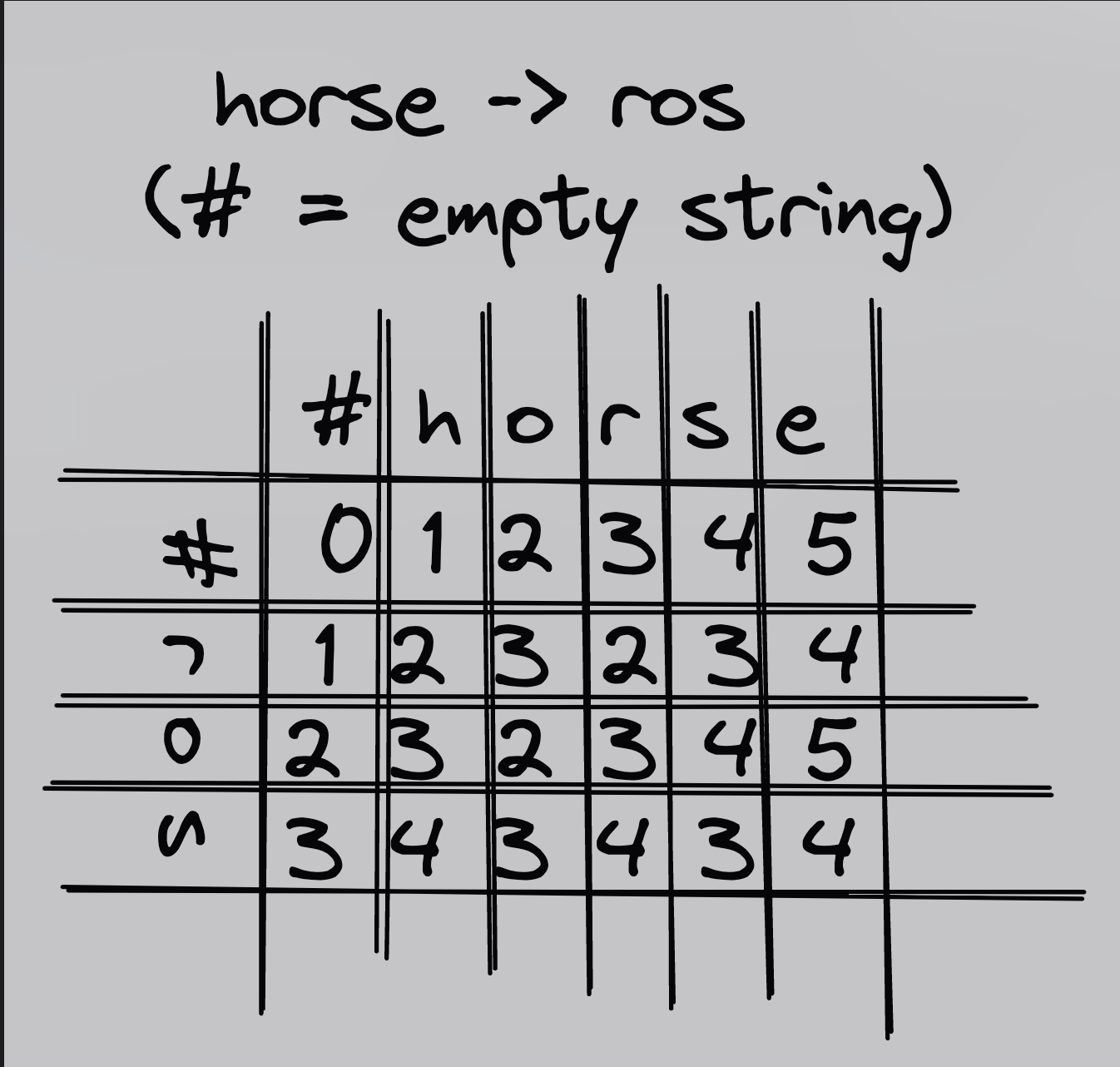# minimum-edit-distance

## A CLI implementing the minimum edit distance algorithm in rust.

## building and running

1. set up rust the raw way or install nix and start a `nix-shell`
2. `cargo run -- --help`

for example: `cargo run -- --from common --to coming --matrix`

You can of course just run the executable directly as well.

## explanation

The minimum edit distance for two strings is the minimum number of insertion, deletion, or replacement operations required to convert the first string to the second. It is generally calculated using a grid, where each value in the cells is the minimum edit distance for the substring that ends at that column and row. For example:

In this case, 4 is the minimum edit distance.

## steps to build the grid

1. first, the initial cells for header column and row (`grid[header_row]` and `grid[first_row]`) represent an empty string, `""`, or `#` in NLP lingo. the cost for these is 0.
2. from the initial 0 cell, for each word, add the accumulating insertion cost for each character (i.e. 1, 2, 3, 4...length of word).
3. for each of the empty cells, the cost of each operation is found in adjascent cells:
if the letters in the columns are the same, just copy the cell labelled "cost of replacement", otherwise pick the cell with the lowest value, add it's associated cost (cost of insert, cost of delete, or or cost of replacement) and use that

Stars: 0
Forks: 0
Issues: 0

### Trending

Contest Algorithms in Rust

A collection of classic data structures and algorithms, emphasizing usability, beauty and clarity over full generality

The Algorithms - Rust

All algorithms implemented in Rust (for educational purposes)

Rust Algorithms and Data structures

Various algorithms and data structures implemented in Rust

rust_algorithms_datastructures

This repository contains implementations of a variety of algorithms and datastructures in Rust

algorithms-by-rust

Implementations of basic algorithms by rust

Implementations of Algorithms and Data Structures in CLRS, as a way to learn Rust and...

Implementations of Algorithms and Data Structures in CLRS, as a way to learn Rust and Algorithms/Data Structures

Experiments with RUST and algorithms

Simple library with tests for next alorithms:

A collection of useful Rust algorithms

Adding Rust Algorithms as a dependency